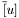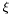#Boost C++ Libraries

...one of the most highly regarded and expertly designed C++ library projects in the world.

This is the documentation for an old version of Boost. Click here to view this page for the latest version.

## Struct template pot_tail_mean_impl

boost::accumulators::impl::pot_tail_mean_impl — Estimation of the (coherent) tail mean based on the peaks over threshold method (for both left and right tails).

## Synopsis

```// In header: <boost/accumulators/statistics/pot_tail_mean.hpp>

template<typename Sample, typename Impl, typename LeftRight>
struct pot_tail_mean_impl {
// types
typedef numeric::functional::average< Sample, std::size_t >::result_type float_type;
typedef float_type                                                       result_type;

// construct/copy/destruct
pot_tail_mean_impl(dont_care);

// public member functions
template<typename Args> result_type result(Args const &) const;
};```

## Description

Computes an estimate for the (coherent) tail mean

Equation 1.10.where,andare the parameters of the generalized Pareto distribution that approximates the right tail of the distribution (or the mirrored left tail, in case the left tail is used). In the latter case, the result is mirrored back, yielding the correct result.

### `pot_tail_mean_impl` public construct/copy/destruct

1. `pot_tail_mean_impl(dont_care);`

### `pot_tail_mean_impl` public member functions

1. `template<typename Args> result_type result(Args const & args) const;`